# 4 November 2014¶

## What are we analysing? An introduction to the Method of Reflections¶

There are two tables below one concerning the origin of the user-"quality" and the other of article-"quality". Let us be clear, "quality" is determined by our exogenous metrics, in this case user-contribution totals, and article-contributor totals (over all *.genius subdomains). When we talk about the origin of the quality, we are discussing which variables we found most important in building a "Method of Reflections" ranking (loosely Google PageRank simulation) of the subdomain network that most closely matches the rankings given by the exogenous.

For instance for the users in lit.genius we find a Spearman $\rho$ correlation of about 0.6. That means we can predict user-contribution activity with a 60% correlation (and probably much higher with full data), just from knowing which users edit which texts (without knowing how much they edit each one).

As fantastical as that sounds, the result comes from an economics insight that the best economies produce the rarest products, and also ubiquitous ones - whereas worse economies produce only ubiquitous products, and hardly ever rare ones. The genius network is similar (see the matrix visualisations below), the top users edit the widest range of texts, and and casual users tend to edit texts that have already been annotated by others.

Going back to the lit.genius example, or method of reflection simulation is dependent on two inputs: $\alpha$, the importance of text-quality, and $\beta$ the importance of user-quality. Of the (25 $\alpha$ values * 25 $\beta$ values)=625 method of reflections simulations, we look for the one with the highest rank correlation, and then consider the $\alpha , \beta$ inputs that got us there (see grid search results below).

Now $\alpha, and \beta$ are negative exponents in our model see equation 13, so the lower or more negative they are the more important they are (think golf). Now if I say that user-quality increases close to linearly, $\beta = 0$, (or in some cases super-linearly $\beta< 0$) with regards to the quality of the editors editing the same texts as you, then we can consider that a "collaborative" subdomain.

Other analyses are available from this data - the importance of neighbouring text quality in predicting user quality, or the importance of neighbouring text quality in predicting text quality. However I argue that the $\beta$ measure for users, is the one that interprets best as collaborativeness.

## Limitations in rank correlations:¶

The rank correlations are important here because they determine the underlying validity of the following results. In general they are lower than the correlations I have encountered on Wikipedia. However they range from about $(0.3, 0.6)$ compare to $(0.5, 0.9)$ on Wikipedia. The results presented here are statistically significant, so I would still consider this analysis valid and meaningful. The reasons this analysis could be lower are: (a) limited data supplied by genius (b) poorer exogenous metrics, IQ may be better or (c) genius users behave differently from other socio-technical websites.

(By the way, I encountered some errors that user_ids, were the same as text_ids. So in this analysis user ids have a prepended "u" and text ids a prepended "t".)

# Results:¶

## Most collaborative¶

Sports, X, and Law, show themselves as the top 3 most collaborative with $\beta$ scores of $-0.72, 0.24, and, 0.4$ respectively . Sports in specific has a negative $\beta$ measure which means that user-quality is predicted super-linearly with regards the neighbouring users. I would predict that there are a group of users that have somehow organised themselves there. The only Wikipedia case found with a negative $\beta$ was US. Military History, which has a dedicated mailing list. Notice also from the text-user matrices that this is one of the tallest matrices, so there are relatively many texts edited per user compared to the others.

X doing very well however is a surprising result to because one would imagine that the "no specific subject nature" might make it more jungle-like and thus less collaborative. The results is actually quite well explained by considering the humorous but true "Zeroeth law of Wikipedia: Wikipedia only works in practice in theory it can never work." Counter-intuitively, people collaborate a better with less constraints rather than more. As people are given more freedoms online they respond well due to unrealised incentives. From an Wikipedian's perspective this is makes a lot of sense, that a company can never make decisions for the community as well as the community.

## Least collaborative¶

News, History, and Rock clock in as the least collaborative of the subdomains with $\beta$ measures of $1.84, 1.52, and, 1.52$. This doesn't mean that they are necessarily "uncollaborative" only less collaborative than the other subdomains. Essentially user-quality is a sub-linear function of the quality of the neighbouring users. Therefore users are not helping one another - as much - to become better users. A naïve explanation might be that since news-commenting and events-comment already have a precedence online for being unproductive pools of back-and-forth argumentation, users unwittingly transfer this behaviour onto the genius website. Even though genius has a different goal to the comment sections of a news websites, users are preconditioned by those sites to not necessarily want to build together an communal annotation.

## Early Conclusions¶

The biggest surprise is that X doing well. This is evidence that an opening up of topics allows users to self-organise better than with a topic constraint. I would present this as push to trusting the user base in defining their own scopes.

# Data Results and Visualisations¶

1. Calibration Data Frames
2. User-Text Matrices
3. Calibration Optimization Grid Searches
4. Example Method of Refections Ranking Convergence
In :
user_df = pd.DataFrame.from_dict(user_calibrations, orient='index')
user_df

Out:
alpha beta rho
history -1.84 1.52 0.481851
law 0.40 0.40 0.429294
lit -0.08 0.88 0.594491
news 0.08 1.84 0.353811
pop -1.68 0.56 0.271077
r-b -1.52 0.56 0.297127
rap -1.84 0.56 0.445590
rock -1.68 1.52 0.476813
screen -2.00 1.36 0.469442
sports -2.00 -0.72 0.307567
tech -2.00 0.88 0.473089
x -2.00 0.24 0.369506
In :
article_df = pd.DataFrame.from_dict(article_calibrations, orient='index')
article_df

Out:
alpha beta rho
history 0.24 -2.00 0.551461
law 0.40 -1.20 0.401671
lit 1.52 -0.08 0.342453
news 0.24 0.56 0.372917
pop 0.08 0.72 0.368729
r-b 0.08 0.72 0.735256
rap 0.40 0.72 0.262893
rock 0.24 -0.24 0.222322
screen 0.08 -0.88 0.434323
sports 0.08 -2.00 0.376457
tech 0.40 0.56 0.660880
x 0.24 -1.52 0.321988
In :
for name, bipartite_dict in bipartite_dicts.iteritems():
M, text_dict, user_dict = viz_bipartite(bipartite_dict, name)

np.save('geniusdata/'+name+'/M.npy', M)
json.dump(text_dict, open('geniusdata/'+name+'/text_dict.json', 'w'))
json.dump(user_dict, open('geniusdata/'+name+'/user_dict.json', 'w'))

text_exogenous_ranks = make_exogenous_ranks(text_dict, all_text_exogenous)
user_exogenous_ranks = make_exogenous_ranks(user_dict, all_user_exogenous)

json.dump(text_exogenous_ranks, open('geniusdata/'+name+'/text_exogenous_ranks.json', 'w'))
json.dump(user_exogenous_ranks, open('geniusdata/'+name+'/user_exogenous_ranks.json', 'w'))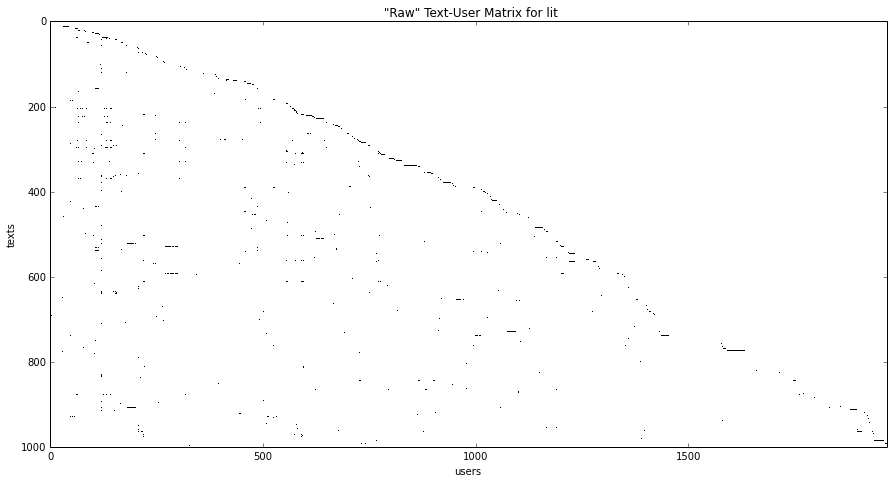<matplotlib.figure.Figure at 0x7f87ef967650>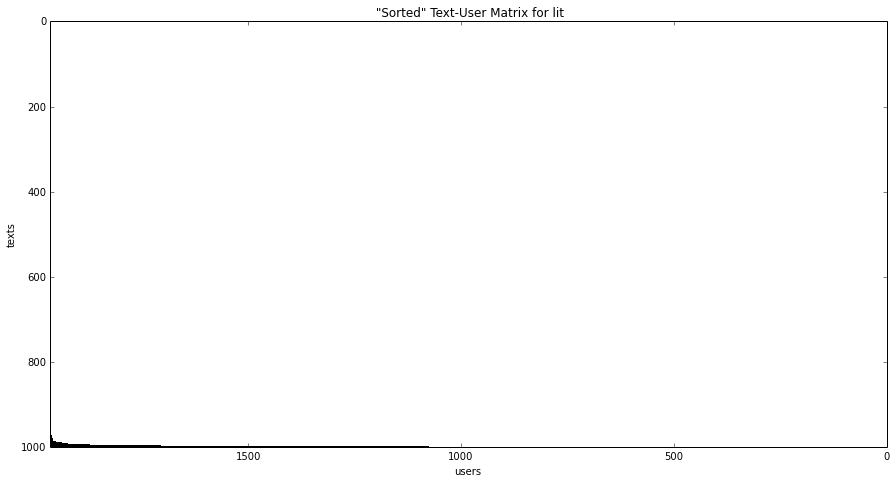<matplotlib.figure.Figure at 0x7f87f10eb510>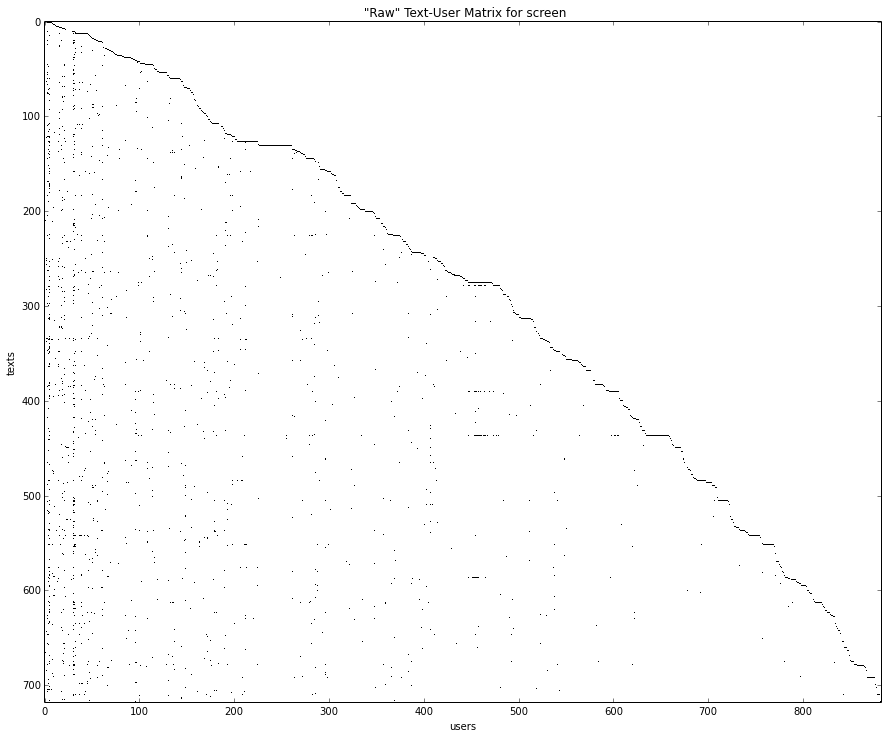<matplotlib.figure.Figure at 0x7f87ef3a0550>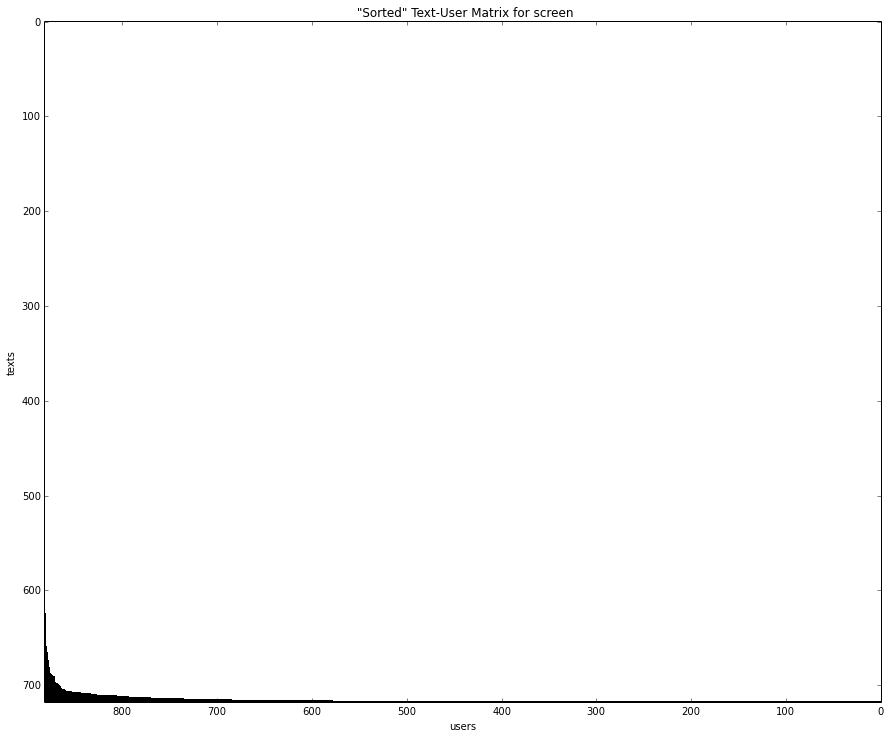<matplotlib.figure.Figure at 0x7f87efd6b2d0>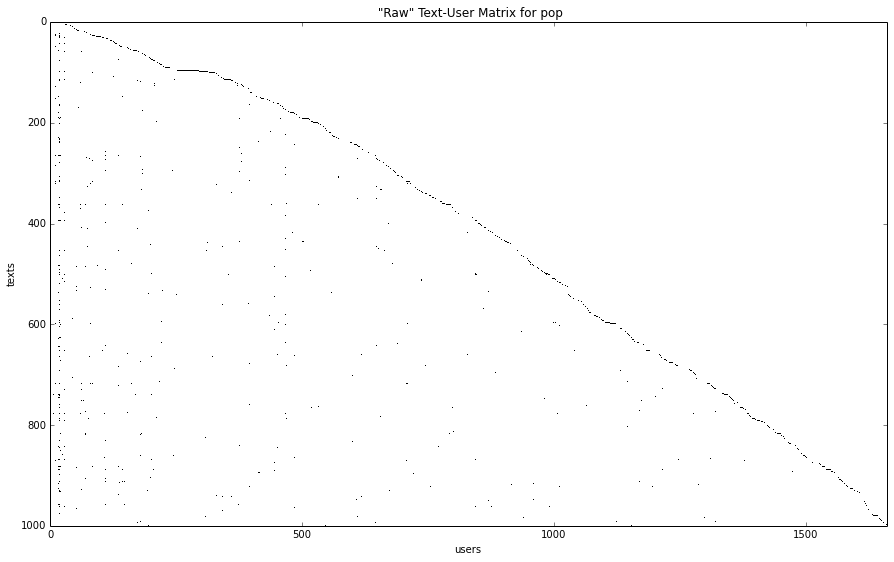<matplotlib.figure.Figure at 0x7f87efb0a150>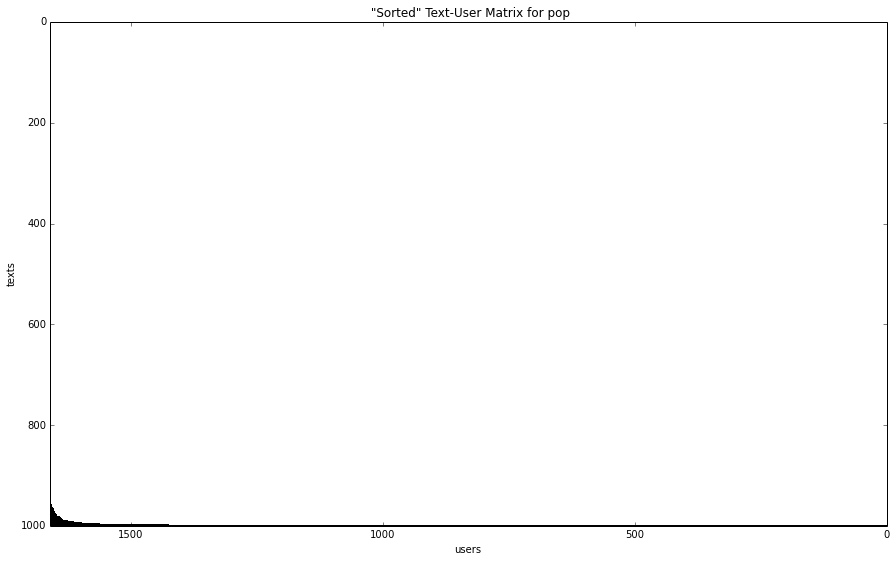<matplotlib.figure.Figure at 0x7f87eeae2190>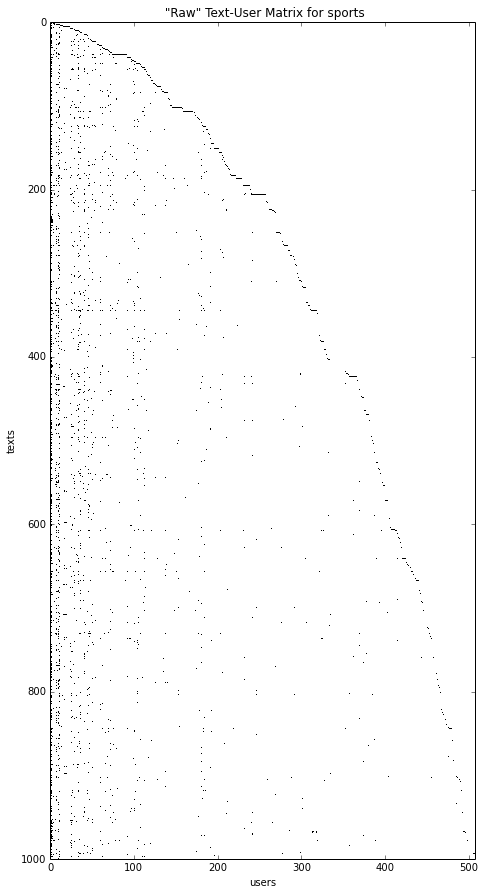<matplotlib.figure.Figure at 0x7f87f0feaa90>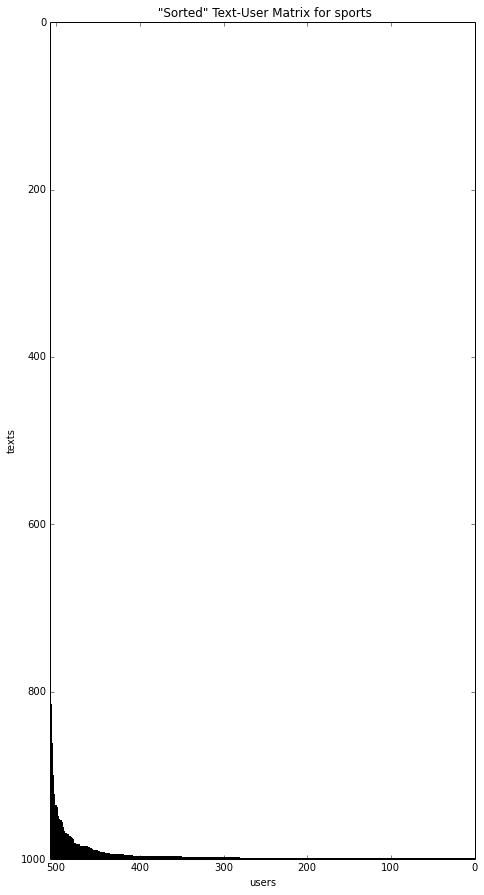<matplotlib.figure.Figure at 0x7f87efae0cd0>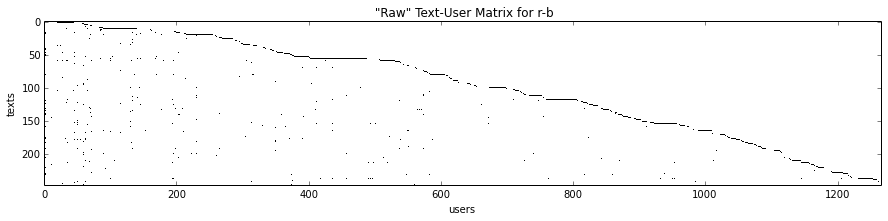<matplotlib.figure.Figure at 0x7f87f10022d0>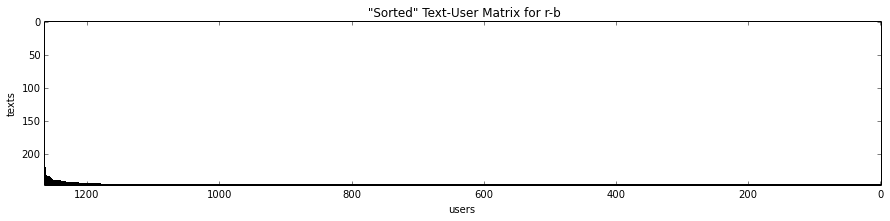<matplotlib.figure.Figure at 0x7f87eda85390>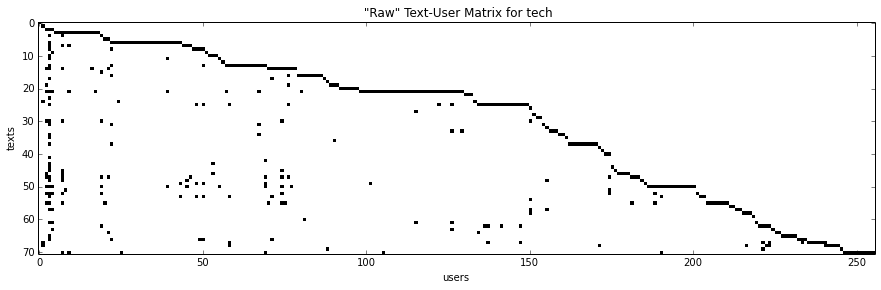<matplotlib.figure.Figure at 0x7f87ebbc7b90>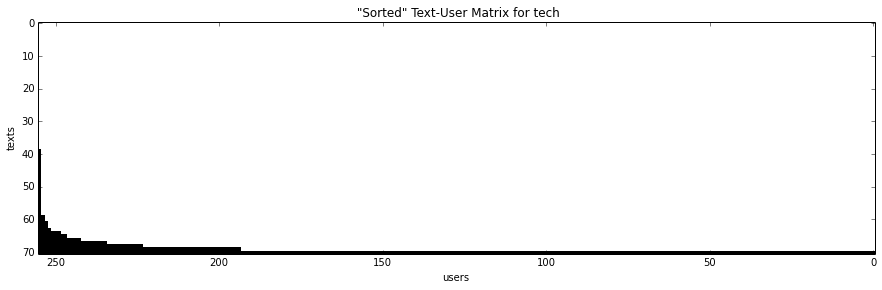<matplotlib.figure.Figure at 0x7f87ef9cbd90>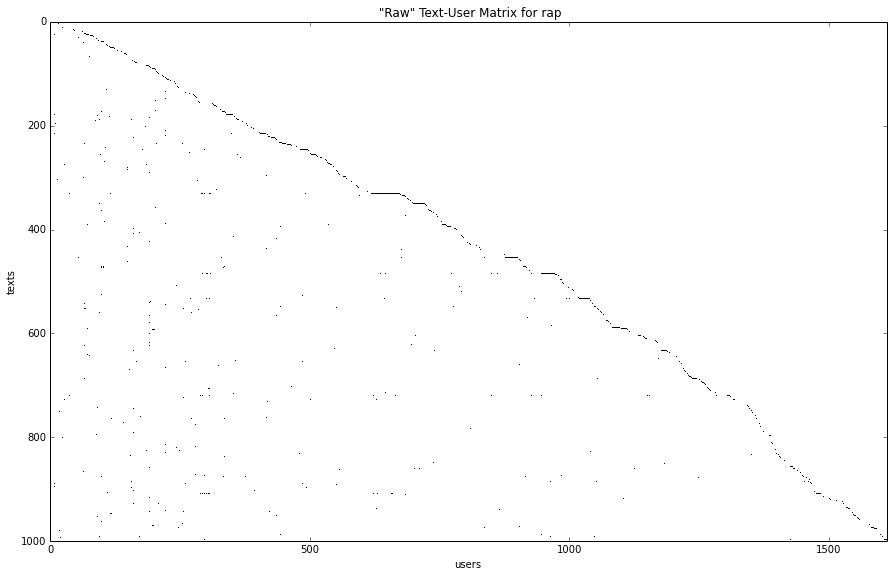<matplotlib.figure.Figure at 0x7f87ef8b2e90>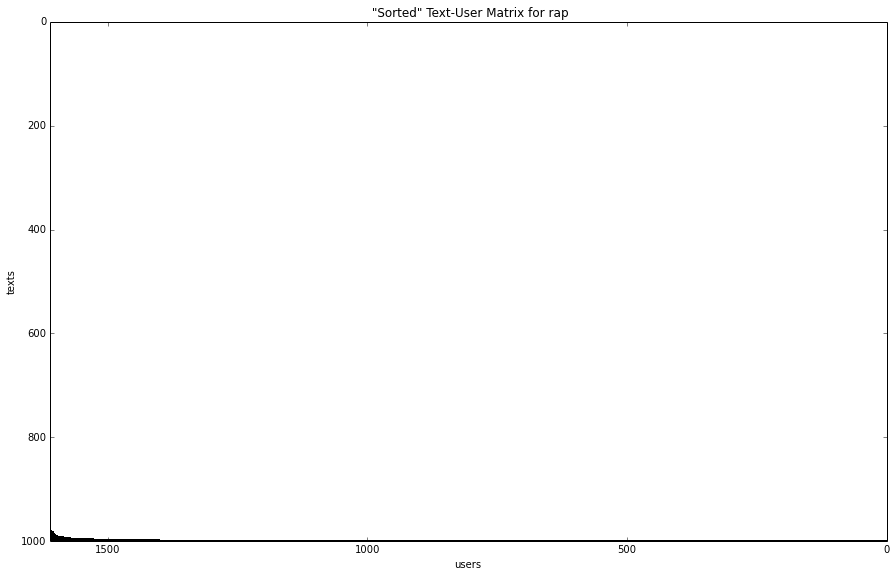<matplotlib.figure.Figure at 0x7f87ecf7c110>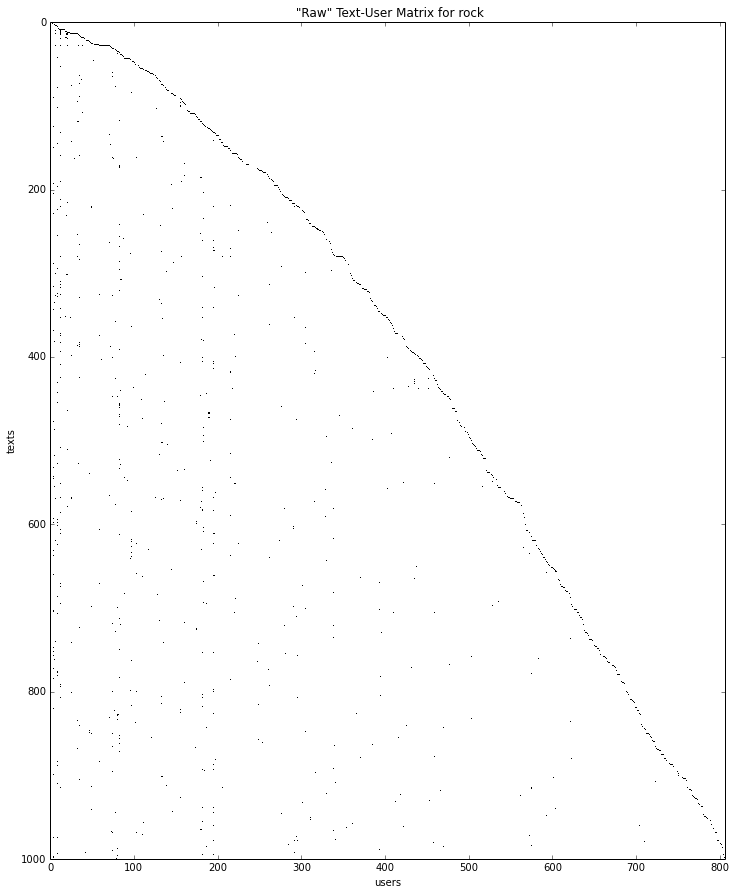<matplotlib.figure.Figure at 0x7f87ed8ea4d0>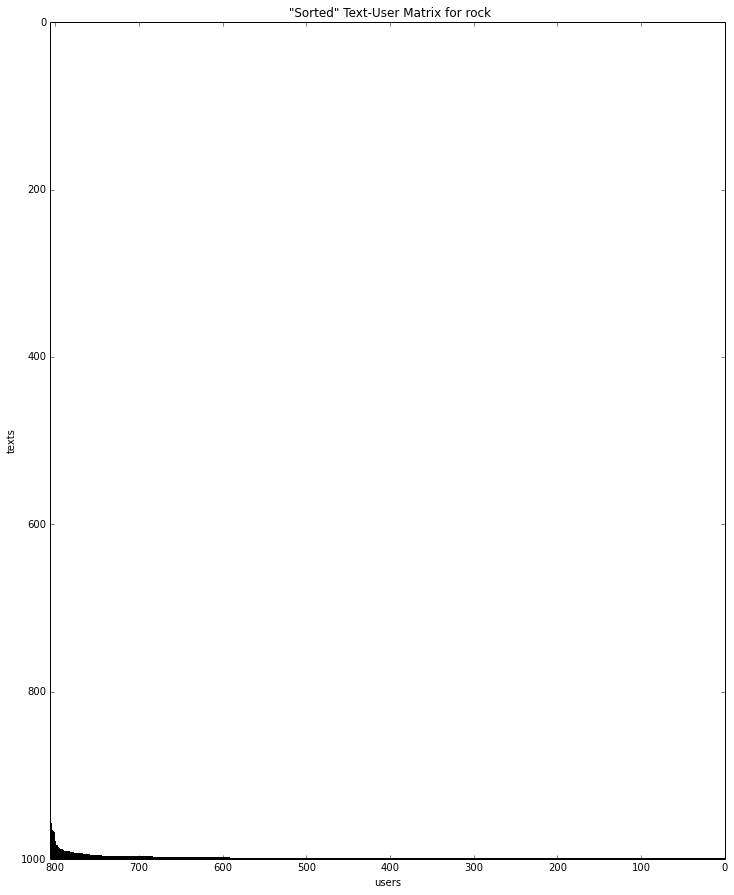<matplotlib.figure.Figure at 0x7f87ef9ba8d0>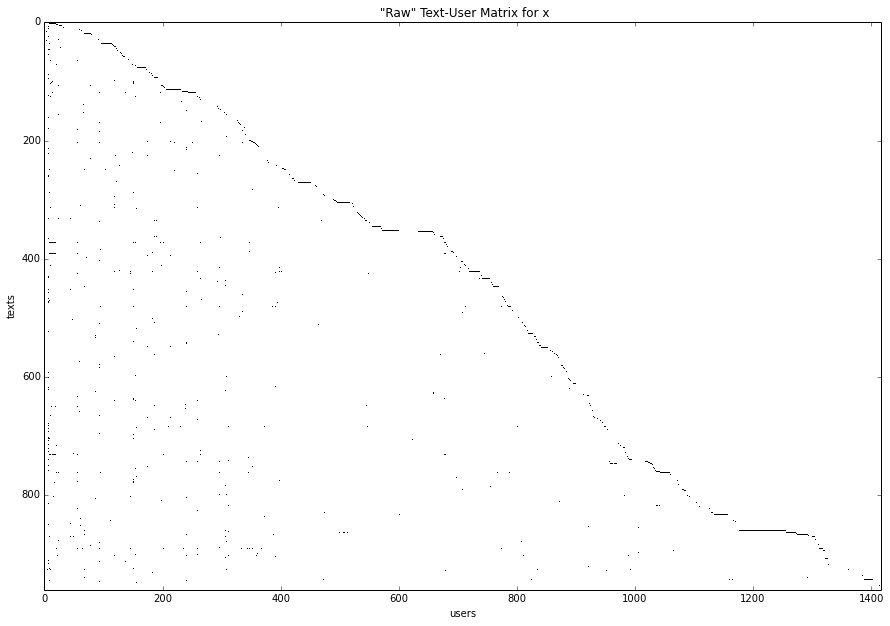<matplotlib.figure.Figure at 0x7f87efb1b690>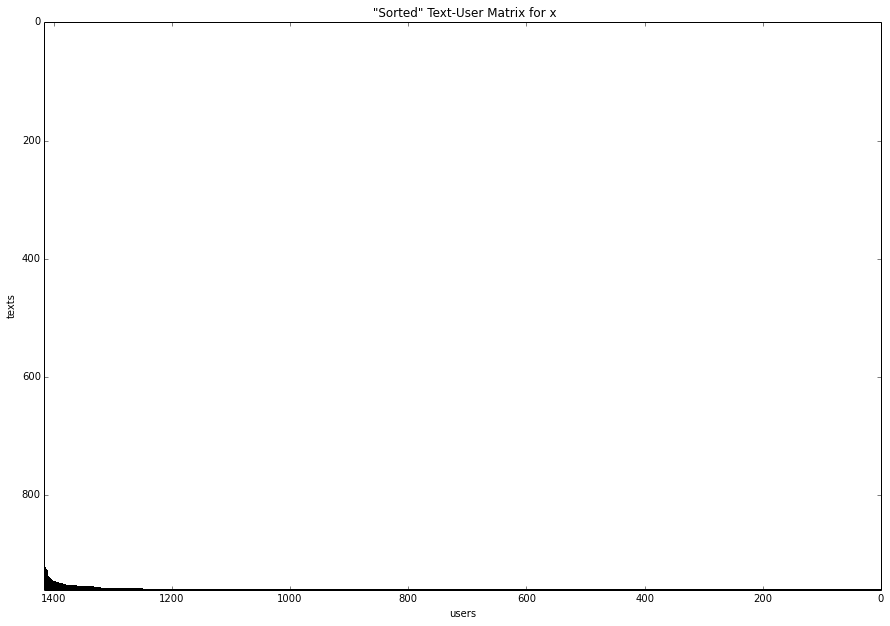<matplotlib.figure.Figure at 0x7f87ebbc7650>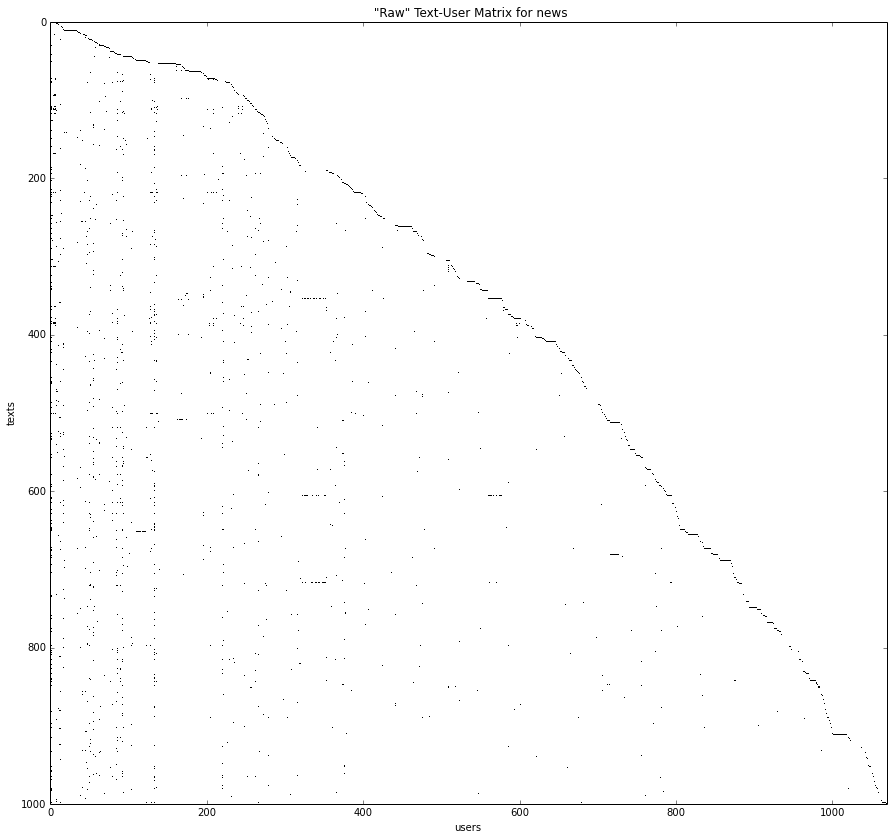<matplotlib.figure.Figure at 0x7f87ef3aa590>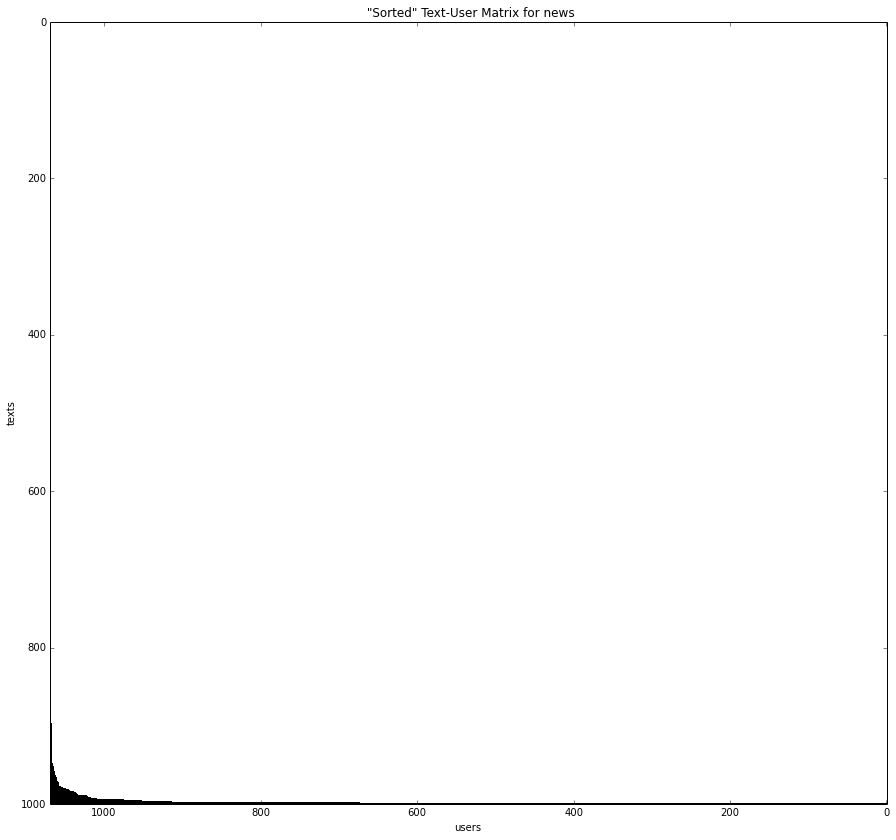<matplotlib.figure.Figure at 0x7f87ef8b2a10>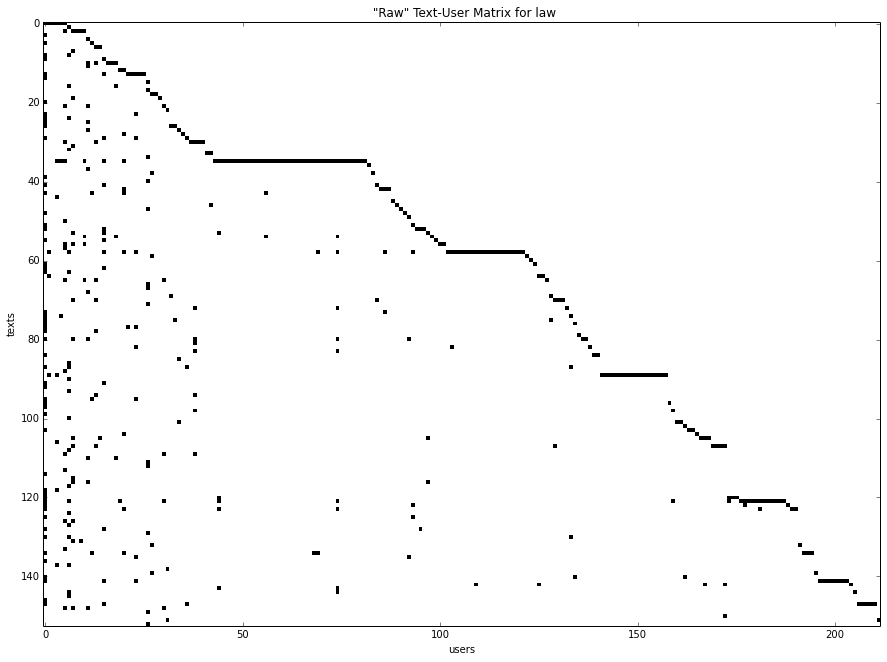<matplotlib.figure.Figure at 0x7f87eec3b5d0>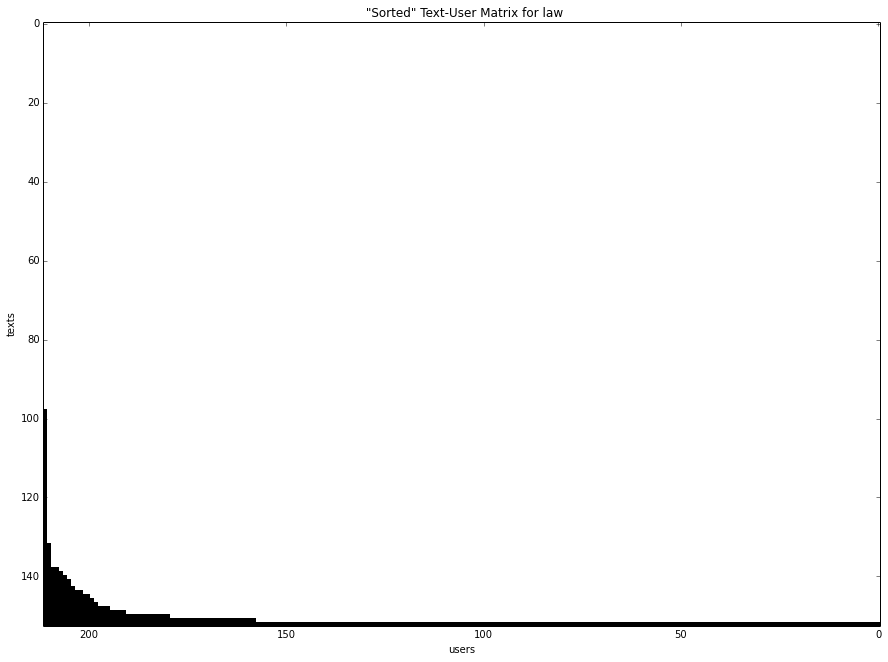<matplotlib.figure.Figure at 0x7f87ef3b4610>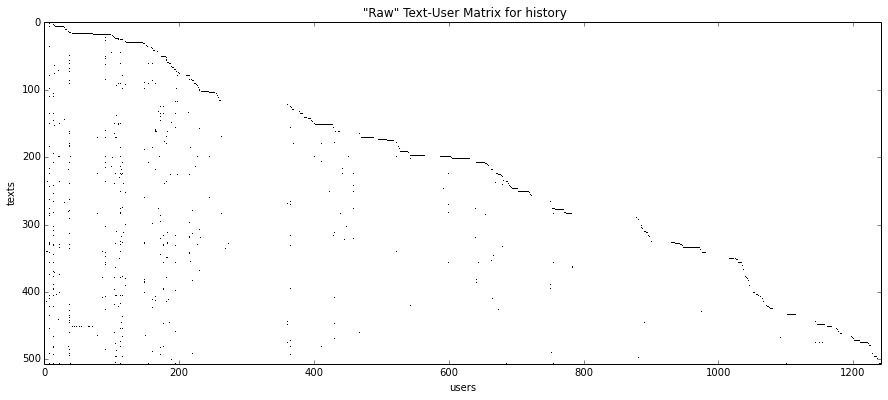<matplotlib.figure.Figure at 0x7f87f0d10310>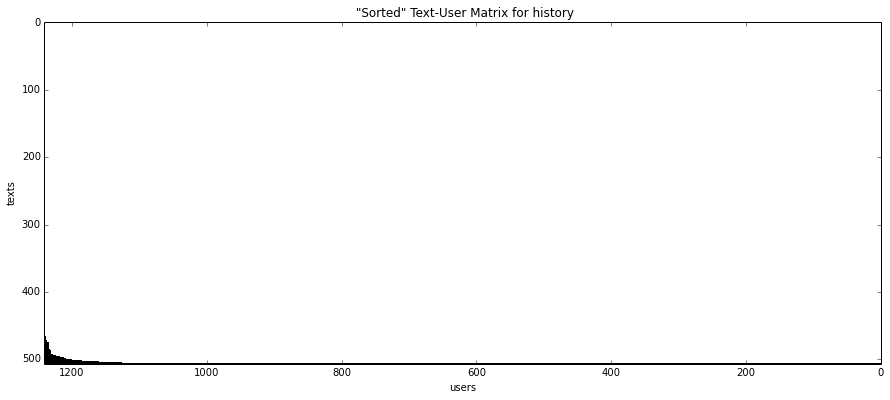<matplotlib.figure.Figure at 0x7f87ed8ea590>
In :
article_calibrations = dict()
for name, data in subdomain_data.iteritems():
calibrations = calibrate(data, 'articles', name)
print name, calibrations
article_calibrations[name] = calibrations

r-b {'alpha': 0.08, 'beta': 0.72, 'rho': 0.73525631322687313}
screen {'alpha': 0.08, 'beta': -0.88, 'rho': 0.43432251032103325}
pop {'alpha': 0.08, 'beta': 0.72, 'rho': 0.3687289516535695}
sports {'alpha': 0.08, 'beta': -2.0, 'rho': 0.37645660335704706}
lit {'alpha': 1.52, 'beta': -0.08, 'rho': 0.34245319801714619}
tech {'alpha': 0.4, 'beta': 0.56, 'rho': 0.6608802421201827}
x {'alpha': 0.24, 'beta': -1.52, 'rho': 0.32198843556375556}
rap {'alpha': 0.4, 'beta': 0.72, 'rho': 0.26289283884165071}
rock {'alpha': 0.24, 'beta': -0.24, 'rho': 0.22232154708118684}
news {'alpha': 0.24, 'beta': 0.56, 'rho': 0.37291663312294737}
law {'alpha': 0.4, 'beta': -1.2, 'rho': 0.40167098257037015}
history {'alpha': 0.24, 'beta': -2.0, 'rho': 0.55146056262305387}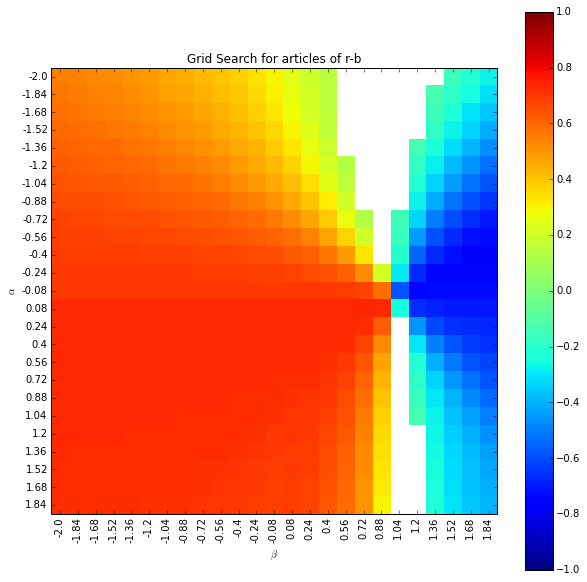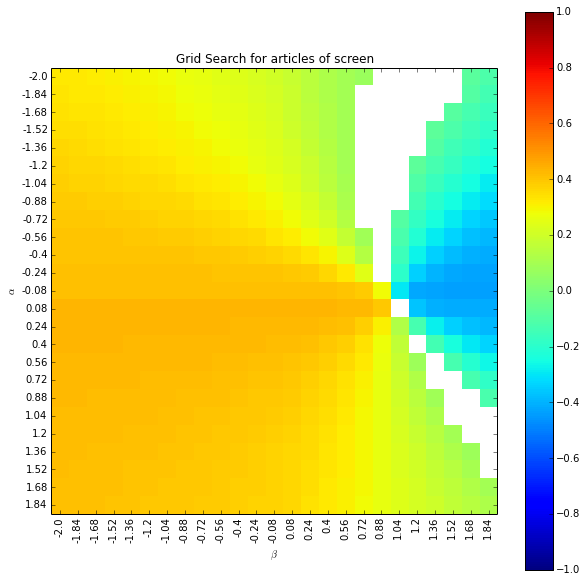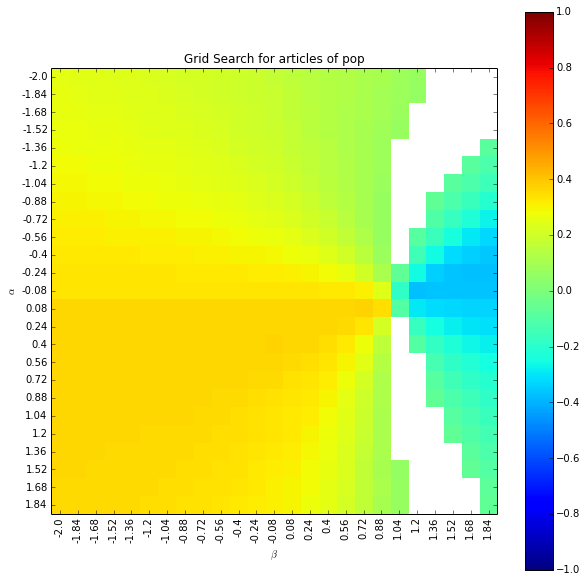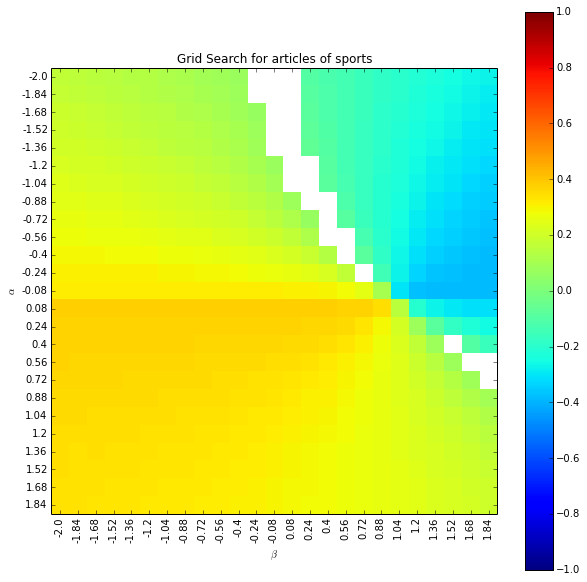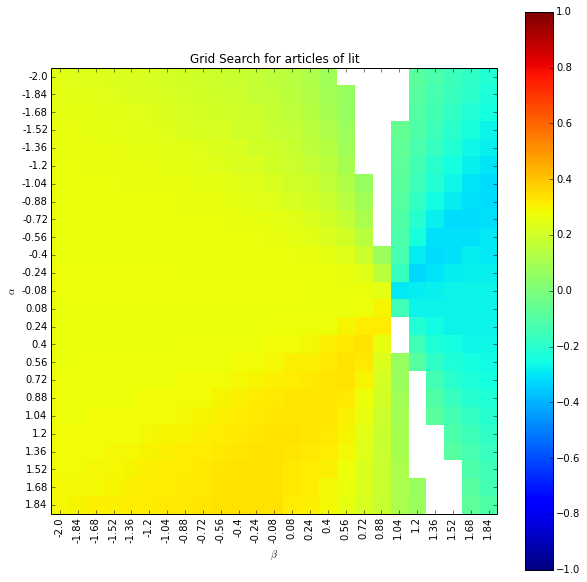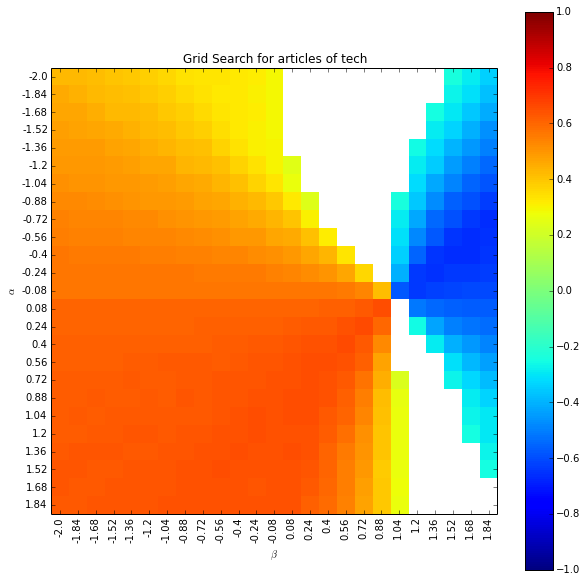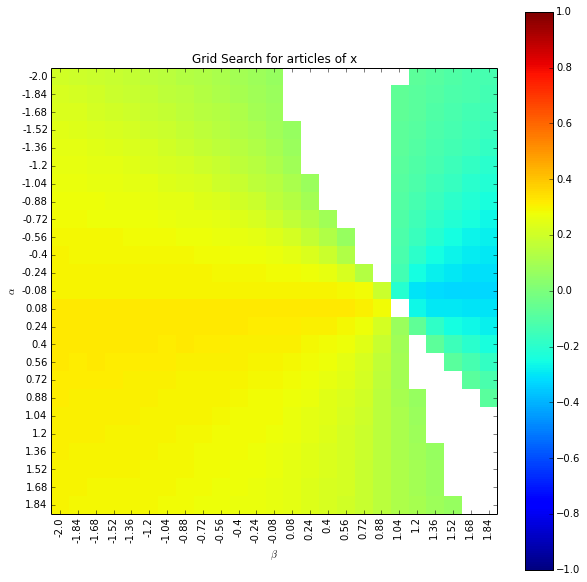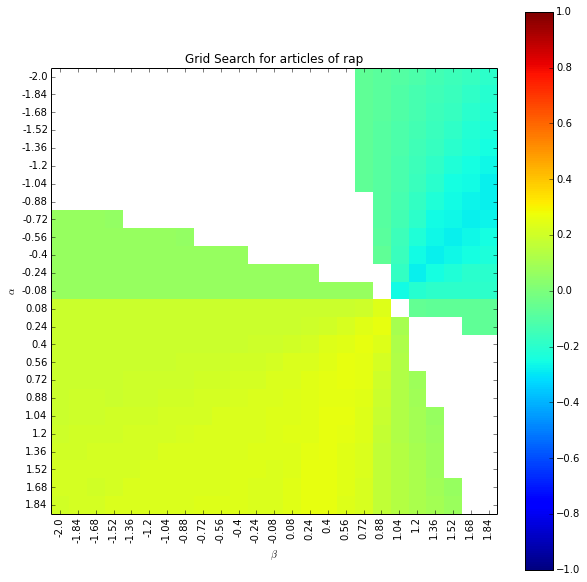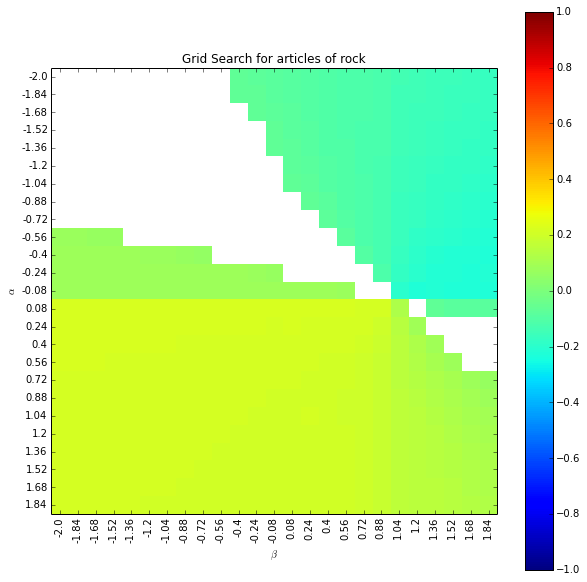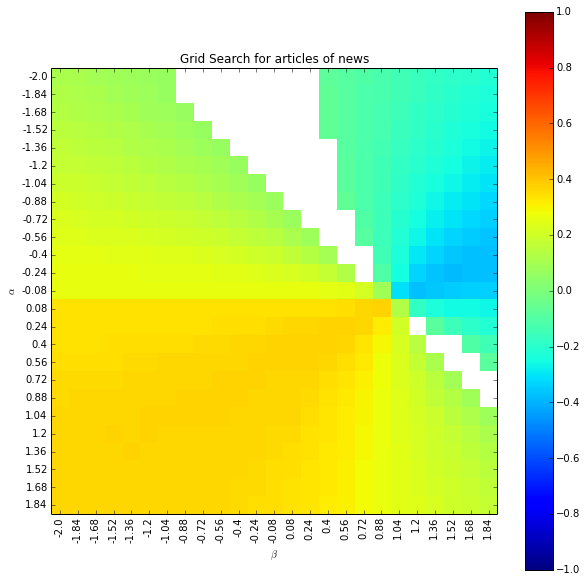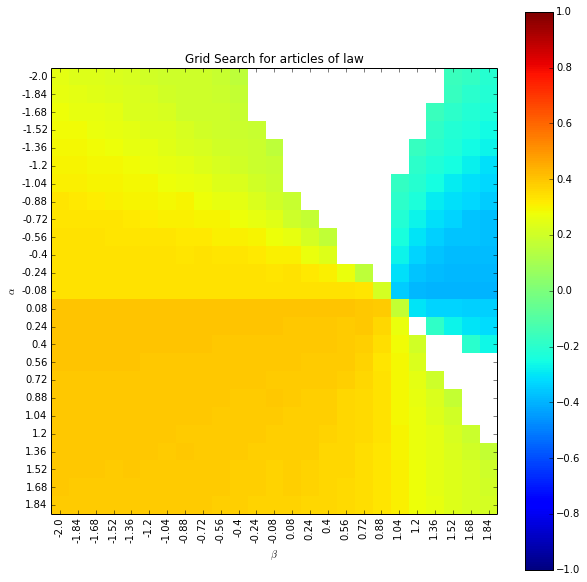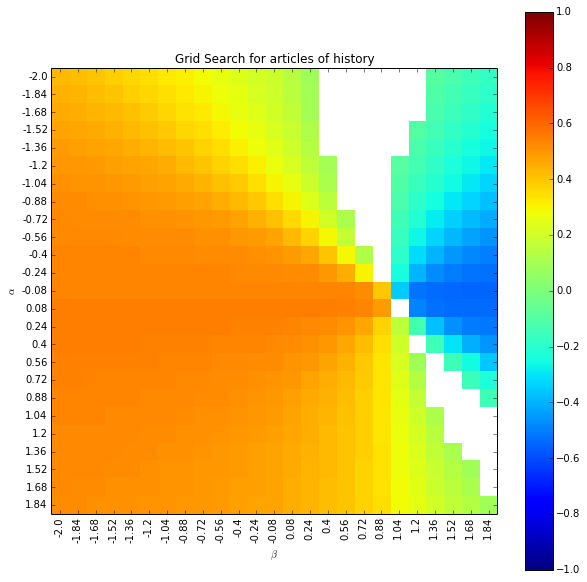In :
user_calibrations = dict()
for name, data in subdomain_data.iteritems():
calibrations = calibrate(data, 'users', name)
print name, calibrations
user_calibrations[name] = calibrations

r-b {'alpha': -1.52, 'beta': 0.56, 'rho': 0.29712697513930469}
screen {'alpha': -2.0, 'beta': 1.36, 'rho': 0.46944201868643853}
pop {'alpha': -1.68, 'beta': 0.56, 'rho': 0.27107665067574438}
sports {'alpha': -2.0, 'beta': -0.72, 'rho': 0.30756706463723915}
lit {'alpha': -0.08, 'beta': 0.88, 'rho': 0.59449131632269803}
tech {'alpha': -2.0, 'beta': 0.88, 'rho': 0.4730886363885195}
x {'alpha': -2.0, 'beta': 0.24, 'rho': 0.36950582819547689}
rap {'alpha': -1.84, 'beta': 0.56, 'rho': 0.44559011704436363}
rock {'alpha': -1.68, 'beta': 1.52, 'rho': 0.47681330924442988}
news {'alpha': 0.08, 'beta': 1.84, 'rho': 0.3538108376542729}
law {'alpha': 0.4, 'beta': 0.4, 'rho': 0.42929372670471688}
history {'alpha': -1.84, 'beta': 1.52, 'rho': 0.48185092927104095}